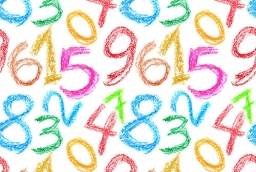# Consecutive 6402

The product of two consecutive natural numbers is 46 less than the product of the other two consecutive natural numbers in a row. Identify unknown numbers.

a =  10
b =  11

### Step-by-step explanation:Did you find an error or inaccuracy? Feel free to write us. Thank you!

Tips for related online calculators
Are you looking for help with calculating roots of a quadratic equation?
Do you have a linear equation or system of equations and looking for its solution? Or do you have a quadratic equation?